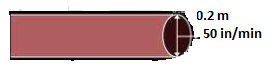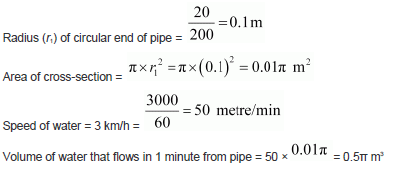Newbie

# A farmer connects a pipe of internal diameter 20 cm from a canal into a cylindrical tank in her field, which is 10 m in diameter and 2 m deep. If water flows through the pipe at the rate of 3 km/h, in how much time will the tank be filled?Q.9

• 0

Today i am solving the ncert class 10 solution  of chapter surface areas and volumes . Find the best solution of the exercise 13.3 question number 9. Give me easiest solution of this question also give me the best solution of this question.A farmer connects a pipe of internal diameter 20 cm from a canal into a cylindrical tank in her field, which is 10 m in diameter and 2 m deep. If water flows through the pipe at the rate of 3 km/h, in how much time will the tank be filled?

Share

1. Consider the following diagram-Volume of water that flows in t minutes from pipe = t×0.5π m3

Volume of water that flows in t minutes from pipe = t×0.5π m3

Radius (r2) of circular end of cylindrical tank =10/2 = 5 m

Depth (h2) of cylindrical tank = 2 m

Let the tank be filled completely in t minutes.

Volume of water filled in tank in t minutes is equal to the volume of water flowed in t minutes from the pipe.

Volume of water that flows in t minutes from pipe = Volume of water in tank

t×0.5π = π×r22×h2

Or, t = 100 minutes

• 0